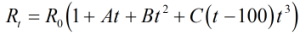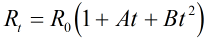# C193 – BS EN 60751 and BS 1904 PRT Calculations

## Description

This calculates the resistance value for a Industrial Platinum Resistance Thermometer at a given temperature (or vice versa).

IEC/BS EN 60751 : Industrial platinum resistance thermometers and platinum temperature sensors (2008)

BS 1904 : Industrial platinum resistance thermometer sensors (1984) (note this standard was superseded by BS EN 60751)

IEC 751(1983)

Kelton calculation reference C193

FLOCALC calculation reference F042

KIMS calculation reference K203

This calculation supercedes C105/K131

## Options

### Standard

• BS EN 60751

Calculation is performed in accordance with standard.

• BS 1904

Calculation is performed in accordance with standard.

### Solve for

• Resistance

Select this option to calculate the equivalent resistance for a given temperature value.

• Temperature

Select this option to calculate the equivalent temperature for a given resistance value.

### Output Type

• Digital

The temperature will be calculated and displayed in the selected temperature unit.

• Analogue

The temperature will be scaled to another unit, current or voltage.

## Constants

Constants as defined in the standard are;

 BS EN 60751 BS 1904 A = 3.9083 x 10-03 °C-1 3.90802 x 10-03 °C-1 B = -5.775 x 10-07 °C-2 -5.802 x 10-07 °C-2 C = -4.183 x 10-12 °C-4 -4.27350 x 10-12 °C-4

## Calculation

All calculations are performed in SI units unless otherwise stated.

### Calculation of resistance

If the temperature is between -200 °C and 0 °C, the resistance of a platinum thermometer Rt is defined as;If the temperature is between 0 °C to 850 °C, the resistance of a platinum thermometer Rt is defined as;Where R0 = The resistance at 0 °C t = The temperature

Typical values for a pt100 are:

 Temperature (°C) Resistance (Ω) 0 100.0000 25 109.7347 50 119.3971 75 128.9874 100 138.5055

### Calculation of temperature

An iterative process is used to calculate the temperature from resistance.